CBSE Sample Question paper - 01 Economics, Class 12

# CBSE Sample Question paper - 01 Economics, Class 12 | Economics Class 12 - Commerce

 1 Crore+ students have signed up on EduRev. Have you?

CBSE SAMPLE PAPER-01
Class – XII Economics

Time : 3 Hours, Maximum Marks : 100
General Instructions:
a. All questions in both sections are compulsory. However, there is internal choice in some
questions.
b. Marks for questions are indicated against each question.
c. Question No.1-5 and 16-20 are very short answer questions carrying 1 mark each. They are
required to be answered in one sentence.
d. Question No.6-8 and 21-23 are short answer questions carrying 3 marks each. Answers to
them should not normally exceed 60 words each.
e. Question No.9-11 and 24-26 are also short answer questions carrying 4 marks each.
Answers to them should not normally exceed 70 words each.
f. Question No.12-15 and 27-30 are long answer questions carrying 6 marks each. Answers to
them should not normally exceed 100 words each
g. Answers should be brief and to the point and the above word limit be adhered to as far as
possible.

Section A: Microeconomics

Q1. If it is given that the total variable cost for producing 15 units of output is Rs. 3000 and for 16 units is Rs. 3,500. Find the value of Marginal Cost.
Ans.
MCn = TVCn –TVCn-1
MC16 = TVC16 – TVC15
= 3,500 – 3,000
= Rs. 500

Q2. Ceteris Paribus, if the government provides subsidies on electricity bills, what would be the likely change in the market demand of desert coolers?
Ans.
Demand for desert coolers will increase.

Q3. Which of the can be referred to as ‘point of satiety’?
i) Marginal Utility is negative
ii) Marginal utility is zero
iii) Total Utility is rising
iv) Total Utility is falling
Ans.
ii) Marginal utility is zero.

Q4. Which of the following is an assumption of Production Possibility Frontier?
i) Resources are not fully employed.
ii) Resources are not equally efficient for production of the two goods.
iii) Resources are not efficiently employed.
iv) Resources available are not fixed.
Ans.
ii) Resources are not equally efficient for production of the two goods.

Q5. State any two central problems under ‘problem of allocation of resources’.
Ans.
a) What to produce and in what quantity?
b) How to produce?
c) For Whom to produce? (Any Two)

Q6. ‘Supply curve is the rising portion of marginal cost curve over and above the minimum of Average Variable cost curve’. Do you agree? Support your answer with valid reason.
Ans
. Yes, we do agree with the given statement that the supply curve is the rising portion of marginal cost curve over and above the minimum of Average Variable cost curve, since no rational producer/seller would like to supply his output to the market if he is unable to recover his per unit variable cost as it would lead to losses between the range of minimum of marginal cost and minimum of average variable cost.

Q7. Explain ‘black marketing’ as a direct consequence of price ceiling.
OR
Explain the concept of ‘buffer stock’ as a tool of price floor.
Ans.
Black marketing may be termed as a direct consequence of price-ceiling, as it implies a situation whereby the commodity under the government’s control policy is illegally sold at a higher price than the one fixed by the government, it may primarily arise due to the presence of consumers who may be willing to pay higher price for the commodity than to go without it.

OR
Buffer stock is an important tool in the hands of government to ensure price floor/minimum support price. If in case the market price is lower than what the government feels should be given to the farmers/producers it would purchase the commodity at higher price from the farmers/producers so as to maintain stock of the commodity with itself to be released in case of shortage of the commodity in future.

Q8. Explain any two sources of restricted entry under monopoly.
Ans.
Sources of restricted entry under monopoly, may be:
iii) Ownership of scarce resource.(Any Two)

Q9. Comment upon the degree of elasticity of demand for Good X, in the following given situations, if the price of the commodity rises from Rs. 5 per unit to Rs. 7 per unit and
the quantity demanded falls from 20 units to 16 units :
i) Using the total household expenditure method,
ii) Using proportionate method.
Ans.
i) P X Q = TE
5 X 20 =  100
7 X 16= 112

Since the price & total expenditure carry positive relation Ed<1, relatively inelastic demand.
ii)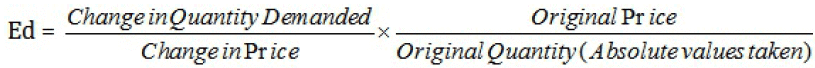=
= 0.5 (Ed<1, relatively inelastic demand.)

Q10. ‘Higher indifference curve represents higher level of satisfaction to the consumer’. Explain the statement, also state the underlying assumption related to this property of
indifference curve.
OR

Q10. A consumer consumes two goods X and Y. Explain what will happen if MUx/Px is greater than MUy/Py?
Ans.
Higher indifference curve represents higher level of satisfaction, in other words any combination that lie on a higher indifference curve i.e. away from origin represents higher level of satisfaction.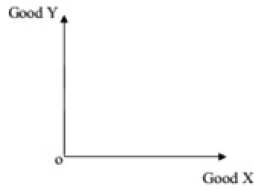Combination B>A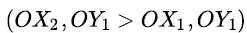The underlying assumption here is the assumption of monotonic preference which represents that a consumer will prefer a combination which contains more of at least one
and no less of the other.
Or
If MUx/Px>MUy/Py, then it means that satisfaction of the consumer derives from spending a rupee on Good X greater than the satisfaction derived from spending a rupee on
Good Y. The consumer will reallocate his income by substituting Good X for Good Y. As the consumption of Good X increases the marginal utility derived from it goes on diminishing and reverse proposition occurs for Good Y, this process will continue till MUx/Px becomes equal to MUy/Py.

Q11. Define Marginal Opportunity Cost. Explain the concept with a hypothetical numerical example.
Ans.
Marginal Opportunity Cost (MOC) of a given commodity along a PPC is defined as the amount of sacrifice of a commodity so as to gain one additional unit of the other
commodity. MOC can also be termed as Marginal Rate of Transformation i.e. the ratio of number of units of a Good sacrificed to produce an additional unit of the other Good.
Schedule.

Q12. a) What is meant by price rigidity, under oligopoly.
b) Elaborate the implication of the conditions of equilibrium of a firm.
Ans.
(a). Price rigidity is the price of the product fixed after deliberations and negotiations by the oligopolistic firms, to which they generally stick with a view to avoid any
sort of price war.
(b). Firm’s equilibrium is that level of output where its profits are maximized Conditions of Firm’s Equilibrium:
i) Marginal Revenue must be equal to Marginal Cost.
ii) Marginal Cost must be rising.
The conditions implies that the slope of rising Marginal Cost Curve is equal to the slope of Marginal Revenue curve.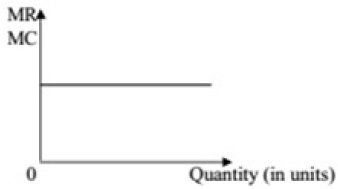Implication of the conditions lies in the fact that beyond the equilibrium point MC would become greater than MR, i.e. for each additional unit sold beyond output OQ the cost of
producing that unit will be more than the revenue generated by the unit.

Q13. a) Distinguish between stock and supply.
b) Complete the following schedule:

 Units Produced TPP(in Rs.) APP(in Rs.) MPP(in Rs.) 1 100 2 140 3 140 4 480

Ans. (a). Stock refers to the total quantity of a commodity available with the seller at any given time. Whereas, Supply refers to that quantity of a commodity which a seller is willing to sell at different prices during a given period of time.
(b).

 Units Produced TPP (in Rs.) APP (in Rs.) MPP (in Rs.

 0 0 - - 1 100 100 100 2 240 120 140 3 420 140 180 4 480 120 60

Q14. Suppose the demand and supply curves of a Commodity-X is given by the following two equations simultaneously:
Qd = 200 – p
Qs = 50 + 2p i)
Find the equilibrium price and equilibrium quantity.
ii) Suppose that the price of a factor of production producing the commodity has changed, resulting in the new supply curve given by the equation
Qs’ = 80 +2p
Analyse the new equilibrium price and new equilibrium quantity as against the original equilibrium price and equilibrium quantity.
Ans.
i) We know that the equilibrium price and quantity are achieved at;
Qd = Qs
200- p =50 + 2p
(-) 3p = (-) 150
Therefore, Equilibrium Price p = 50
And, Equilibrium Quantity q = 200 – 50 = 150 units

ii) If the price of factor of production has changed, then under the new conditions;
Qd = Qs
200- p = 80 + 2p
(-) 3p = (-) 120
Therefore, Equilibrium Price p = 40
And, Equilibrium Quantity q = 200 – 40 = 160 units
Thus as the equilibrium price is decreasing the equilibrium quantity is increased.

Q15. Show diagrammatically the conditions for consumer ’s equilibrium, in Hicksian analysisof demand.
Ans.
In the Hicksian/ Indifference Curve analysis, a consumer attains equilibrium when
i)Budget line is tangential to the Indifference curve at a unique combination of two goods. i.e, Slope of Indifference Curve = Slope of Budget line or MRSxy = (-) Px/Py
ii) Indifference Curve is strictly convex to origin at the point of tangency i.e., MRSxy must be diminishing.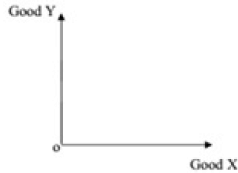Section B: Macroeconomics

Q16. If an economy is to control recession like most of the Euro-Zone nations, which of the following can be appropriate:
i) Reducing Repo Rate
ii) Reducing CRR
iii) Both (i) and (ii)
iv) None of (i) and (ii)
Ans.
(iii) Both (i)and (ii)

Q17. Which of the following agency is responsible for issuing Rs.1 currency note in India?
i) Reserve Bank of India.
ii) Ministry of Commerce
iii) Ministry of finance
iv) Niti Aayog
Ans.
(iii)Ministry of finance

Q18. Flow of Goods & services and factors of production across different sectors in a barter economy is known as:
i) Circular flow
ii) Real flow
iii) Monetary Flow
iv) Capital Flow
Ans.
(ii) Real flow

Q19. The government budget of a hypothetical economy presents the following information, which of the following value represents Budgetary Deficit. (all fig. in Rs. crores)
A. Revenue Expenditure = 25,000
B. Capital Receipts = 30,000
C. Capital Expenditure = 35,000
D. Revenue Receipts = 20,000
E. Interest Payments = 10,000
F. Borrowings = 20,000
i) Rs. 12,000
ii) Rs. 10,000
iii) Rs. 20,000
iv) None of these
Ans.
(iv) None of the above.
Budgetary Deficit = Revenue expenditure + Capital Expenditure-(Revenue receipts+ Capital
receipts)
= 25000+ 35000-(20000+3000)
=Rs. 50,000 crores

Q20. Which of the following statement is true?
i) Loans from IMF is a Revenue Receipt.
ii) Higher revenue deficit necessarily leads to higher fiscal deficit.
iii) Borrowing by a government represents a situation of fiscal deficit.
iv) Revenue deficit is the excess of capital receipts over the revenue receipts.
Ans.
(iii) Borrowing by a government represents a situation of fiscal deficit.

Q21. ‘Devaluation and Depreciation of currency are one and the same thing’. Do you agree? How do they affect the exports of a country?
Ans.
*Devaluation is the fall in the value of domestic currency in relation to foreign currency as planned by the government in a situation when exchange rate is not determined by the forces of demand & supply but is fixed by the government of different countries where as Depreciation is the fall in the value of domestic currency in relation to foreign currency in a situation when exchange rate is determined by the forces of demand & supply in the international money market.
As a general phenomena, any depreciation/devaluation of currency may result into increase in exports of the goods and services from the country since it would increase the global competiveness of the goods.

Q22. If in an economy Saving function is given by S = (-) 50 + 0.2 Y and Y = Rs. 2000 crores; consumption expenditure for the economy would be Rs. 1,650 crores and the
autonomous investment is Rs. 50 crores and the marginal propensity to consume is 0.8. True or False?

Or
“Economists are generally concerned about the rising Marginal Propensity to Save (MPS) in an economy”. Explain why?

Ans. Yes all the given values are correct
S= -50+0.2Y
S= -50+.02(2000)
=-50 +400
= 350 crores
At equilibrium level of income: Y = C + S
2,000 = C + 350
C = 2000 – 350 = 1,650 (in Rs. crores)
MPC + MPS = 1
MPC + 0.2 = 1
MPC = 1-0.2 = 0.8
OR
Since the sum of MPC and MPS is unity any increase in Marginal Propensity to Save (MPS) would directly lead to decrease in Marginal Propensity to Consume (MPC). This means that may lead to lesser proportion of the additional income going to consumption which is a vital factor of Aggregate Demand/Expenditure. This may further lead to fall in equilibrium level of income in the economy.

Q23. Explain how the economy achieves equilibrium level of income using Savings- Investment (S-I) approach.
Ans.
As per the S-I approach equilibrium if achieved where ex-ante Savings are equal to exante investments. Savings and investments indicate leakages and injections respectively, thus at equilibrium the leakages and injections are equal to each other.

Q24. Suppose in an imaginary economy GDP at Market Price in a particular fiscal year was Rs. 4,000 crores, National Income was Rs. 2,500 crores, Net Factor Income paid by the economy to Rest of the World was Rs. 400 crores and the value of Net Indirect Taxes is Rs. 450 Crores. Estimate the value of consumption of fixed capital for the economy from the given data.
Ans.
NNPfc = GDPmp – Consumption of fixed capital – Net factor income to abroad – Net
indirect taxes
2500 = 4000-CFC -450 -400
2500 = 3150 – CFC
CFC = 650 (in Rs. crores)

Q25. What is meant by ‘official reserve transactions’? Discuss their importance in
Balance of Payments.
Ans.
Transactions by a central bank that cause changes in its official reserves. These are usually purchases or sales of its own currency in the exchange market in exchange for foreign currencies or other foreign-currency- denominated assets. They may be Autonomous Receipts and Autonomous Payments, disequilibrium between which may occur as deficit/surplus in balance of payment.

Q26. State the various components of the Expenditure Method that are used to calculate national income.
OR
Discuss any two differences between GDP at constant prices and GDP at current Prices.
Ans.
Components of Expenditure method:-
(a) Private Final Consumption Expenditure
(b) Government Final Consumption Expenditure
(c) Investment Expenditure
(d) Net Exports
Or
Two main difference between GDP at current prices and at constant price are:
1. GDP at current prices are measured at Current Year’s Prices whereas GDP at constant prices are measured at base year’s prices.
2. GDP at current prices may increase even if there is no flow of goods and services whereas GDP at constant prices will only increase when there is an increase in the flow of goods and services.

Q27. “Governments across nations are too much worried about the term fiscal deficit”. Do you think that fiscal deficit is necessarily inflationary in nature? Support your answer with valid reasons.
Ans.
The term fiscal deficit is the difference between the government's total expenditure and its total receipts (excluding borrowing). Such borrowings are generally financed by issuing new currency which may lead to inflation, however, if the borrowings are for the infrastructural developmental purposes this may lead to capacity building and may not be inflationary.

Q28. Derive a straight line saving curve using the following consumption function:
C = 20 + 0.6Y.
Presuming the income levels to be Rs. 100, Rs. 200 and Rs. 300 crores. Also calculate that level of income where consumption is equal to income.
For Visually Impaired candidates: Calculate savings from the following saving function:
C = 20 + 0.6Y.
Presuming the income levels to be Rs. 100, Rs. 200 and Rs. 300 crores. Also calculate that level of income where consumption is equal to income.
Ans.
C = 20 + 0.6Y

 Y C S 100 80 20 200 140 70 300 200 100

Properly Labeled Diagram

Q29. a) What is meant by Repo Rate? How does the Central Bank use this measure to control inflationary conditions in an economy?
b) What is meant by Margin Requirement? How does the Central Bank use this measure to control deflationary conditions in an economy?
Ans.

a) Repo rate is the rate of interest at central bank lends money to commercial banks for a short term. The central bank fixes the Repo Rate and it plays the role of an indicator of lending rate and deposit rate fixation by the banks. Under inflationary conditions central bank increases the Repo Rate.

b) Marginal requirement refers to the difference between market value of the security offered for loans and the amount of loans offered by the commercial banks. The
central bank fixes the margin requirements and under deflationary conditions central bank reduces the margin requirements.

Q30. Compute (a) Domestic Income and (b) Net National Disposable Income.

 S.No. Items Amount(in Rs.) i) Net Exports 155 ii) Government final consumption expenditure 2500 iii) Subsidies 120 iv) Gross domestic fixed capital formation 1190 v) Net factor income to abroad 125 vi) Net decrease in inventories 100 vii) Net Exports (-) 420 viii) Net Indirect Taxes 470 ix) Net Current transfers from abroad 350 x) Current replacement cost 145 xi) Private final consumption expenditure 2200

OR

Explain any four limitations of using GDP as a measure/index of welfare of a country.
Ans.

Domestic Income = xi + ii + iv – vi + vii – x – viii
= 2200 + 2500 + 1190 – 100 – 420 – 145 – 470
= 4755 (in Rs. crores)
Net National Disposable Income = National Income + Net Indirect Taxes + Net Current
Transfers from ROW
=Domestic Income - v + viii+ ix
= 4755 -125+470+350
= 5450 (in Rs. crores)
OR
Four limitations of using GDP as a measure/index of welfare of a Country are:

I. Distribution of GDP
II. Composition of GDP
III. Non-Monetary Exchanges
IV. Externalities.

The document CBSE Sample Question paper - 01 Economics, Class 12 | Economics Class 12 - Commerce is a part of the Commerce Course Economics Class 12.
All you need of Commerce at this link: Commerce

## Economics Class 12

196 videos|244 docs|77 tests

## Economics Class 12

196 videos|244 docs|77 tests

Track your progress, build streaks, highlight & save important lessons and more!

,

,

,

,

,

,

,

,

,

,

,

,

,

,

,

,

,

,

,

,

,

,

,

,

;